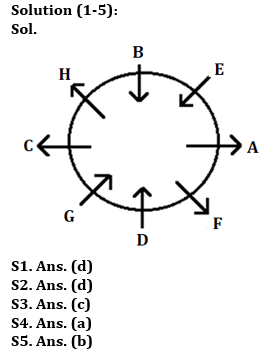Latest Banking jobs   »

# Reasoning Quiz For Bank of Baroda AO 2023 -17th May

Directions (1-5): Study the following information carefully and answer the given questions.
Eight students have seated around a circular table in which some are facing towards the centre and some are facing outside the centre. E sits third to the right of D. Only two persons sit between G and B and both are facing same direction. A sits third to the right of the one who sits second to the left of G. F is neither immediate neighbour of G nor B. C sits third to the right of F who sits opposite to H. G is facing same direction as D but opposite to C and A. E sits second to the right of H who is facing same direction as F. B and D faces each other. E faces towards the center.

Q1. Who among the following sits third to the right of A?
(a) E
(b) F
(c) D
(d) G
(e) None of these

Q2. Who among the following sits opposite to C?
(a) B
(b) E
(c) H
(d) A
(e) None of these

Q3. How many persons sits between D and H, when counting left of D?
(a) Four
(b) Three
(c) Two
(d) One
(e) None

Q4. What is the position of B with respect to F?
(a) Third to the left
(b) Immediate right
(c) Second to the right
(d) Immediate Left
(e) None of these

Q5. How many persons facing inside?
(a) One
(b) Four
(c) Three
(d) Two
(e) None of these

Directions (6-10): In these questions, relationship between different elements is shown in the statements. These statements are followed by two conclusions:
(a) If only conclusion I follows.
(b) If only conclusion II follows.
(c) If either conclusion I or II follows.
(d) If neither conclusion I nor II follows.
(e) If both conclusion I and II follow.

Q6. Statements: A ≥ Q, U > V = R, A > B ≤ V, U < S
Conclusion: I. B < S II. R < S

Q7. Statements: D ≥ Q, P > J, O > Q > J, T > D
Conclusion: I. O > T II. T > J

Q8. Statements: A ≥ Q, U > V = R, A > B ≤ V, U < S
Conclusion: I. Q ≥ U II. U > Q

Q9. Statements: R ≥ Z, C = B < Z, D ≥ R > O
Conclusion: I. D > B II. O < C

Q10. Statements: Q = B, K < E < B, J ≥ E, R ≤ Q
Conclusion: I. J > K II. R ≤ B

SolutionsSolution (6-10):
S6. Ans.(e)
Sol. I. B < S (True) II. R < S (True)

S7. Ans.(b)
Sol. I. O > T (False) II. T > J (True)

S8. Ans.(c)
Sol. I. Q ≥ U (False) II. U > Q (False)

S9. Ans.(a)
Sol. I. D > B (True) II. O < C (False)

S10. Ans.(e)
Sol. I. J > K (True) II. R ≤ B (True)## FAQs

### What is the selection process of Bank of Baroda AO 2023?

The selection process of Bank of Baroda AO consists of Online exam and Interview

#### Congratulations!Union Budget 2023-24: Free PDF